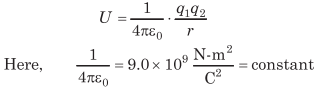# What is Energy in Physics | Definition, Formula, Types, Units – Work, Energy and Power

Energy Definition Physics:
The energy of a body is its capacity of doing work. It is a scalar quantity.

We are giving a detailed and clear sheet on all Physics Notes that are very useful to understand the Basic Physics Concepts.

## What is Energy in Physics | Definition, Formula, Types, Units – Work, Energy and Power

SI unit of energy:
SI unit is joule

CGS unit of energy:
CGS unit is erg.

Energy Dimensional Formula:
Dimensional formula is [ML²T-2].

Types of Energy:
There are several types of energies, such as

• Mechanical Energy
• Kinetic Energy
• Potential Energy
• Chemical Energy
• Light Energy
• Heat Energy
• Sound Energy
• Nuclear Energy
• Electric Energy

Mechanical Energy Definition:
The sum of kinetic and potential energy is known as mechanical energy.

Mechanical Energy Types:
1. Kinetic Energy Definition:
The energy possessed by any object by virtue of its motion is called its kinetic energy.
Kinetic energy of an object is given by K = $$\frac{1}{2}$$ mv² = $$\frac{p^{2}}{2 m}$$
where, m = mass of the object, v = velocity of the object and p = mv = momentum of the object.

2. Potential Energy Definition:
The energy possessed by any object by virtue of its position or configuration is called its potential energy.
In one dimensional motion, potential energy U(x) is defined if force F(x) can be written as
F(x) = $$-\frac{d U}{d x}$$
or
F(x) . dx = -dU
or
$$\int_{x_{i}}^{x_{f}} F(x) \cdot d x=-\int_{U_{i}}^{U_{f}} d U=U_{i}-U_{f}$$

• Potential energy is defined only for conservative forces. It does not exist for non-conservative forces.
• Potential energy depends upon frame of reference.

Types of Potential Energy
There are three important types of potential energies.

1. Gravitational Potential Energy
2. Elastic Potential Energy
3. Electric Potential Energy

(i) Gravitational Potential Energy:
If a body of mass m is raised through a height h against gravity, then its gravitational potential energy = mgh.

(ii) Elastic Potential Energy:
If a spring of spring constant k is stretched through a distance x, then elastic potential energy of the spring
= $$\frac{1}{2}$$ kx².The variation of potential energy with distance is shown in figure.

(iii) Electric Potential Energy:
The electric potential energy of two point charges q1 and q2 separated by a distance r in vacuum is given byEquilibrium
If the forces acting an the object are conservative and it is in equilibrium, then

Fnet = 0 ⇒ $$\frac{-d U}{d r}$$ = 0 or $$\frac{d U}{d r}$$ = 0

Types of Equilibrium Physics
Equilibrium of an object or system can be divided into three types
(i) Stable equilibrium Physics:
An object is said to be in stable equilibrium, if on slight displacement from equilibrium position, it has the tendency to come back.
Here, $$\frac{d^{2} U}{d r^{2}}$$ = positive

(ii) Unstable equilibrium Physics:
An object is said to be in unstable equilibrium, if on slight displacement from equilibrium position, it moves in the direction of displacement.
Here, $$\frac{d^{2} U}{d r^{2}}$$ = negative

(iii) Neutral equilibrium Physics:
An object is said to be in neutral equilibrium, if on displacement from its equilibrium position, it has neither the tendency to move in direction of displacement nor to come back to equilibrium position.
Here, $$\frac{d^{2} U}{d r^{2}}$$ = 0

Work-Energy Theorem:
Work done by a force in displacing a body is equal to change in its kinetic energy.where, Ki = initial kinetic energy
and Kf = final kinetic energy.

Regarding the work-energy theorem, it is worth noting that
(i) If Wnet is positive, then Kf – Ki= positive, i.e. Kf > Ki or kinetic energy will increase and vice-versa.

(ii) This theorem can be applied to non-inertial frames also. In a non-inertial frame it can be written as Work done by all the forces (including the Pseudo force) = Change in kinetic energy in non-inertial frame.

Other Forms of Energy
Heat Energy Definition:
A body possess heat energy due to the disorderly motion of its molecules. Heat energy is also related to the internal energy of the body.

Chemical Energy Definition:
Chemical energy is stored in the chemical bonds of atoms and molecules. If the total energy of the reactant is more than the product of the reaction, then heat is released and the reaction is said to be an exothermic reaction. If the reverse is true, then heat is absorbed and the reaction is endothermic.

Electrical Energy Definition:
It is the energy which is associated with the flow of electric current or with charging or discharging of a body.

Nuclear Energy Definition:
It is the binding energy of the nucleus of an atom. It is used in nuclear reactors, nuclear fission etc.

Mass-Energy Equivalence:
According to Einstein, the mass can be transformed into energy and vice-versa. When Δm mass disappears, then produced energy,
E = Δmc²
where, c is the speed of light in vacuum.

Work, Energy and Power:
Work, energy and power are the three quantities which are inter-related to each other. The rate of doing work is called power. An equal amount of energy is consumed to do a work. So, basically the power is the rate at which energy is consumed to complete a work.